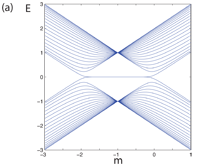July 2011
Mon Tue Wed Thu Fri Sat Sun
« Jun   Aug »
123
45678910
11121314151617
18192021222324
25262728293031

## Absence of topological insulator phases in non-Hermitian PT-symmetric Hamiltonians

Yi Chen Hu, Taylor L. HughesIn this work we consider a generalization of the symmetry classification of topological insulators to non-Hermitian Hamiltonians which satisfy a combined PT-symmetry (parity and time-reversal). We show via examples, and explicit bulk and boundary state proofs that the typical paradigm of forming topological insulator states from Dirac Hamiltonians is not compatible with the construction of non-Hermitian PT-symmetric Hamiltonians. The topological insulator states are PT-breaking phases and have energy spectra which are complex (not real) and thus such non-Hermitian Hamiltonians are not consistent quantum theories.

http://arxiv.org/abs/1107.1064
Other Condensed Matter (cond-mat.other); Quantum Physics (quant-ph)

## PT symmetry in relativistic quantum mechanics

Carl M. Bender, Philip D. Mannheim

In nonrelativistic quantum mechanics and in relativistic quantum field theory, time t is a parameter and thus the time-reversal operator T does not actually reverse the sign of t. However, in relativistic quantum mechanics the time coordinate t and the space coordinates x are treated on an equal footing and all are operators. In this paper it is shown how to extend PT symmetry from nonrelativistic to relativistic quantum mechanics by implementing time reversal as an operation that changes the sign of the time coordinate operator t. Some illustrative relativistic quantum-mechanical models are constructed whose associated Hamiltonians are non-Hermitian but PT symmetric, and it is shown that for each such Hamiltonian the energy eigenvalues are all real.

http://arxiv.org/abs/1107.0501
High Energy Physics – Theory (hep-th); Mathematical Physics (math-ph); Quantum Physics (quant-ph)